# GED Math : Operations with Negative Numbers

## Example Questions

← Previous 1 3 4 5 6

### Example Question #1 : Operations With Negative Numbers

Solve: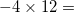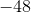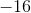Explanation:

When a negative number is multipied by a positive number, the result is negative.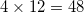Therefore,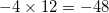.

### Example Question #1 : Operations With Negative Numbers

Evaluate: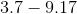Do not use a calculator.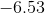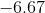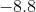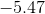Explanation:

To subtract a larger positive number from a smaller positive number, do the reverse, then affix a negative symbol, as demonstrated here: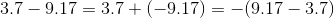So subtract 3.7 from 9.17: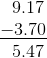Since the answer must be negative, it is.

### Example Question #2 : Operations With Negative Numbers

Evaluate: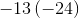Do not use a calculator.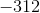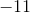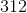Explanation:

To obtain the product of two numbers of unlike sign, multiply their absolute values: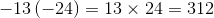The product will remain positive.

### Example Question #4 : Operations With Negative Numbers

Evaluate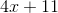for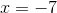. Do NOT use a calculator.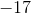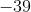Explanation:

Substituteforin the expression.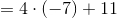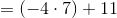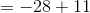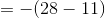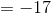### Example Question #1 : Operations With Negative Numbers

Evaluate: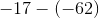Do not use a calculator.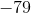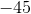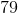Explanation:

To subtract with negative numbers, change to an addition and make the second number its opposite: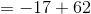To add two numbers of unlike sign, if the positive has the greater absolute value, simply subtract the absolute values.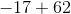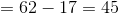### Example Question #3 : Operations With Negative Numbers

Evaluate: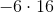Do NOT use a calculator.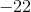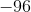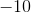Explanation:

To obtain the product of two numbers of unlike sign, multiply their absolute values and affix a negative symbol.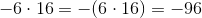### Example Question #3 : Operations With Negative Numbers

Which of these is not equal to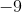?

Do not use a calculator.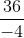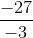The reciprocal of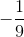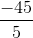Explanation:

Only one of the choices is positive -, which is the quotient of two negative numbers. This must be the correct response.

### Example Question #4 : Operations With Negative Numbers

Evaluate: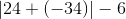Explanation: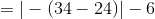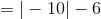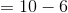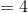### Example Question #2 : Operations With Negative Numbers

How many of these statements are correct?

I)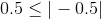II)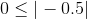III)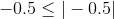One

None

Three

Two

Three

Explanation:

The absolute value of a negative number can be determined by removing the negative symbol, so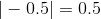. Of the three numbers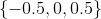, all are less than or equal to 0.5.

### Example Question #10 : Operations With Negative Numbers

Raiseto the third power.

Do not use a calculator.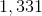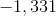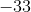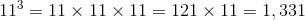The result must be negative, so the correct response is.